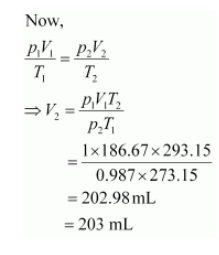# The drain cleaner, Drainex contains small bits of aluminum which react with caustic soda to produce dihydrogen.

Question:

The drain cleaner, Drainex contains small bits of aluminum which react with caustic soda to produce dihydrogen. What volume of dihydrogen at 20 °C and one bar will be released when 0.15g of aluminum reacts?

Solution:

The reaction of aluminium with caustic soda can be represented as:

$2 \mathrm{Al}+2 \mathrm{NaOH}+2 \mathrm{H}_{2} \mathrm{O} \longrightarrow 2 \mathrm{NaAlO}_{2}+3 \mathrm{H}_{2}$

$2 \times 27 \mathrm{~g}$

$3 \times 22400 \mathrm{~mL}$

At STP (273.15 K and 1 atm), 54 g (2 × 27 g) of Al gives 3 × 22400 mL of H2..

$\therefore 0.15 \mathrm{~g}$ Al gives $\frac{3 \times 22400 \times 0.15}{54} \mathrm{~mL}$ of $\mathrm{H}_{2}$ i.e., $186.67 \mathrm{~mL}$ of $\mathrm{H}_{2}$

At STP,

$p_{1}=1 \mathrm{~atm}$

$V_{1}=186.67 \mathrm{~mL}$

$T_{1}=273.15 \mathrm{~K}$

Let the volume of dihydrogen beat p2 = 0.987 atm (since 1 bar = 0.987 atm) and T2 = 20°C = (273.15 + 20) K = 293.15 K..Therefore, 203 mL of dihydrogen will be released.